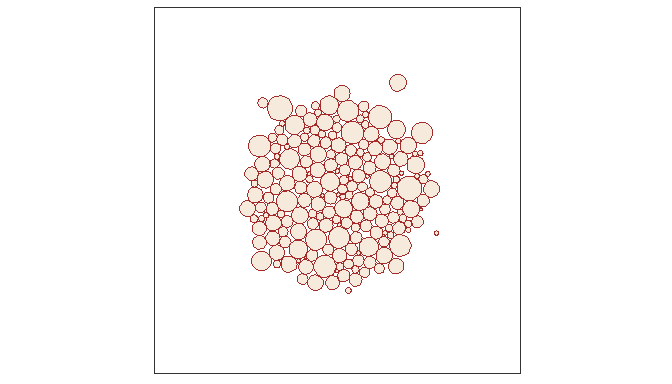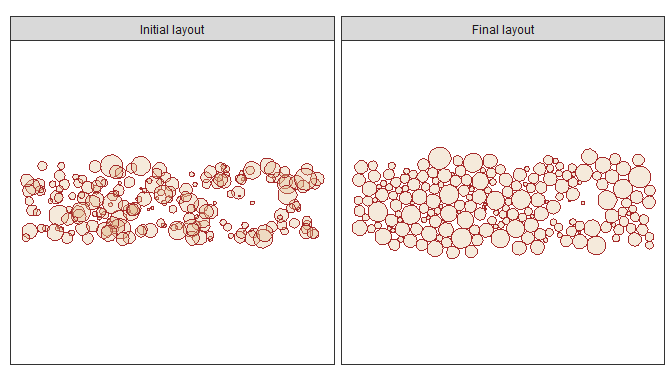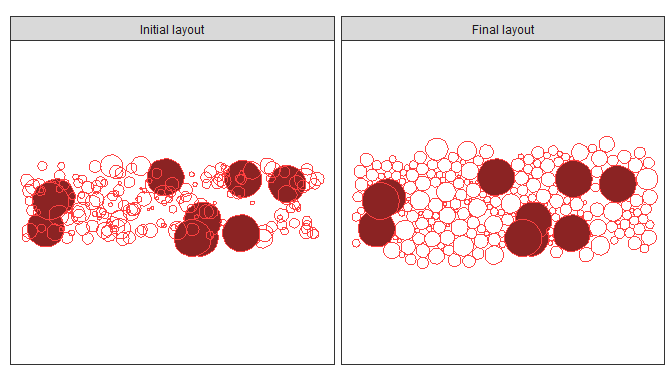# An introduction

## Circle packing algorithms

A large number of circle packing algorithms, both deterministic and stochastic, have been developed. This package provides several of the simpler ones from which you can choose.

• circleRepelLayout searches for a non-overlapping layout by iteratively moving circles through pair-wise repulsion. Circle positions are constrained to remain within a rectangular area (although this can be made infinite for unconstrained search). To avoid edge effects, the bounding area can be treated as a torus so that, for example, a circle pushed over the left-hand edge will re-enter the bounding area at the right-hand edge. This is a very simple and fairly inefficient algorithm but often produces good results.

• circleProgressiveLayout consecutively places circles so that each is externally tangent to two previously placed circles. This algorithm is deterministic, although different layouts can be produced by changing the order of the input circles. It is also very efficient and, as such, suitable for working with large data sets. See its vignette for examples.

• circleGraphLayout attempts to find an arrangement that satisfies an input graph of adjacencies. The implementation in this package is experimental. See its vignette for details.

## A first example

We will start by creating a set of circles of various sizes and then using the circleRepelLayout function to find a non-overlapping arrangement which we can display with ggplot.

First we create a set of random circles, positioned within the central portion of a bounding square, with smaller circles being more common than larger ones. We will express circle size as area. This is the default for circleRepelLayout but you can also express size as radius and specify sizetype = “radius” when calling the function.

set.seed(42)

ncircles <- 200
limits <- c(-40, 40)
inset <- diff(limits) / 3
maxarea <- 40

areas <- rbeta(ncircles, 1, 5) * maxarea

Next, we use the circleRepelLayout function to try to find a non-overlapping arragement, allowing the circles to occupy any part of the bounding square. The returned value is a list with elements for the layout and the number of iterations performed.

library(packcircles)

res <- circleRepelLayout(areas, xlim = limits, ylim = limits)

cat(res$niter, "iterations performed") ## 375 iterations performed The layout is returned as a data frame with circle centre coordinates and radii. head( res$layout )
##            x           y    radius
## 1 13.1147122  -0.6669234 1.1308380
## 2  0.4995586 -18.3833175 0.9218804
## 3 -1.6417293   8.6140819 1.0971732
## 4  4.2994181 -21.5244595 1.5616752
## 5 -3.6841895  -1.2990119 0.4189622
## 6 14.8024399  -9.1574151 1.5501321

We convert this to a data set of circle vertices, suitable for display with ggplot. The number of vertices can be controlled with the npoints argument but we use the default.

The resulting data set has an integer id field which corresponds to the position or row of the circle in the original data passed to circleRepelLayout.

dat.gg <- circleLayoutVertices(res$layout, sizetype = "radius") head(dat.gg) ## x y id ## 1 14.24555 -0.6669234 1 ## 2 14.21002 -0.3856954 1 ## 3 14.10567 -0.1221380 1 ## 4 13.93906 0.1071885 1 ## 5 13.72065 0.2878748 1 ## 6 13.46416 0.4085675 1 And now we can draw the layout. library(ggplot2) t <- theme_bw() + theme(panel.grid = element_blank(), axis.text=element_blank(), axis.ticks=element_blank(), axis.title=element_blank()) theme_set(t) ggplot(data = dat.gg, aes(x, y, group = id)) + geom_polygon(colour="brown", fill="burlywood", alpha=0.3) + coord_equal(xlim=limits, ylim=limits)## Specifying initial circle positions In the previous example, where we passed a vector of circle sizes to circleRepelLayout, the function randomly assigned starting positions to the circles by placing them close to the centre of the bounding area. Alternatively, we can specify the initial positions beforehand. To illustrate this, we will initially position all of the circles near one corner of the bounding area. dat.init <- data.frame( x = runif(ncircles, limits, limits), y = runif(ncircles, limits, limits) / 4.0, area = areas ) res <- circleRepelLayout(dat.init, xlim = limits, ylim = limits, xysizecols = 1:3) cat(res$niter, "iterations performed")
## 184 iterations performed

Next we display the initial and final layouts with ggplot. Notice that in our initial layout we expressed circle sizes as areas so we need to specify that when calling the circleLayoutVertices function, otherwise it assumes sizes are radii.

# Get vertex data for the initial layout where sizes are areas
dat.gg.initial <- circleLayoutVertices(dat.init, sizetype = "area")

# Get vertex data for the layout returned by the function where
# sizes are radii
dat.gg.final <- circleLayoutVertices(res$layout) dat.gg <- rbind( cbind(dat.gg.initial, set=1), cbind(dat.gg.final, set=2) ) ggplot(data = dat.gg, aes(x, y, group = id)) + geom_polygon(colour="brown", fill="burlywood", alpha=0.3) + coord_equal(xlim=limits, ylim=limits) + facet_wrap(~ set, labeller = as_labeller(c(1 = "Initial layout", 2 = "Final layout")))## Moving and fixed circles The circleRepelLayout function accepts an optional weights argument to give extra control over the movement of circles at each iteration of the layout algorithm. The argument takes a numeric vector with values in the range 0-1 inclusive (any values outside this range will be clamped to 0 or 1). A weight of 0 prevents a circle from moving at all while a weight of 1 allows full movement. To illustrate this, we will choose a few circles from the data set used ealier, make them larger and fix their position by setting their weights to 0.0. # choose several arbitrary circles and make them the larger # than the others largest.ids <- sample(1:ncircles, 10) dat.init$area[largest.ids] <- 2 * maxarea

# re-generate the vertex data for the initial circles, adding a column
# to indicate if a circle is fixed (the largest) or free
dat.gg.initial <- circleLayoutVertices(dat.init, sizetype = "area")

dat.gg.initial$state <- ifelse(dat.gg.initial$id %in% largest.ids, "fixed", "free")

# now re-run the layout algorithm with a weights vector to fix the position
# of the largest circle
wts <- rep(1.0, nrow(dat.init))
wts[ largest.ids ] <- 0.0

res <- circleRepelLayout(dat.init, xlim = limits, ylim = limits, weights=wts)

dat.gg.final <- circleLayoutVertices(res$layout) dat.gg.final$state <- ifelse(dat.gg.final\$id %in% largest.ids, "fixed", "free")

dat.gg <- rbind(
cbind(dat.gg.initial, set = 1),
cbind(dat.gg.final, set = 2)
)

ggplot(data = dat.gg, aes(x, y, group=id, fill=state)) +

geom_polygon(colour="brown1", show.legend = FALSE) +

scale_fill_manual(breaks = c("fixed", "free"), values=c("brown4", NA)) +

coord_equal(xlim=limits, ylim=limits) +

facet_wrap(~ set,
labeller = as_labeller(c(1 = "Initial layout",
2 = "Final layout")))Notice that fixed circles that were overlapping in the initial layout are still overlapping in the final layout. Setting their weights to 0.0 resulted in them being ignored by circleRepelLayout`.